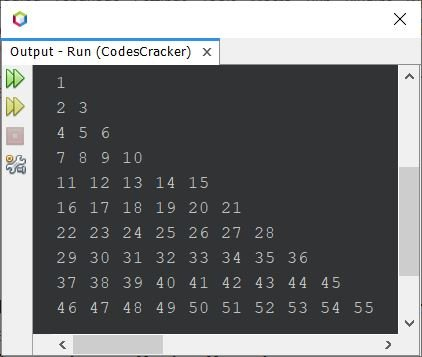# Java Program to Print Floyd's Triangle

This post covers a program in Java that prints Floyd's triangle. A right-angled triangle formed using natural numbers can be called as a Floyd's triangle. For example:

```1
2 3
4 5 6
7 8 9 10
11 12 13 14 15 ```

is Floyd's triangle of 5 rows or lines.

## Print Floyd's Triangle in Java using for Loop

The question is, write a Java program to print Floyd's triangle. The program given below is its answer:

```public class CodesCracker
{
public static void main(String[] args)
{
int row=10, i, j, num=1;
for(i=0; i<row; i++)
{
for(j=0; j<=i; j++)
{
System.out.print(num+ " ");
num++;
}

System.out.print("\n");
}
}
}```

The snapshot given below shows the sample output produced by above Java program on printing of a Floyd's triangle, that are of 10 rows:## Print Floyd's Triangle in Java using while Loop

Now let me create the same program using while loop, to show you, how the same program can be written or replaced using while.

```public class CodesCracker
{
public static void main(String[] args)
{
int row=10, i=0, j, num=1;
while(i<row)
{
j=0;
while(j<=i)
{
System.out.print(num+ " ");
num++;
j++;
}

System.out.print("\n");
i++;
}
}
}```

The above program can also be created in this way:

```public class CodesCracker
{
public static void main(String[] args)
{
int row=10, i=0, j, num=1;
while(i++ < row)
{
j=0;
while(j++ <= i)
System.out.print(num++ + " ");
System.out.print("\n");
}
}
}```

You'll still get the exact output as of previous program. But the program becomes shorter than previous. The ++ operator after the variable, indicates that the operator is post-increment operator. Post-increment operator, first uses the current value of the variable, then increments its value by one, after the use.

## Print Floyd's Triangle of Given Size in Java

Here is one more program on Floyd's triangle. This program allows user to define the size or row size of Floyd's triangle:

```import java.util.Scanner;

public class CodesCracker
{
public static void main(String[] args)
{
int num=1;
Scanner s = new Scanner(System.in);

System.out.print("Enter the Row Size: ");
int row = s.nextInt();

for(int i=0; i<row; i++)
{
for(int j=0; j<=i; j++)
{
System.out.print(num+ " ");
num++;
}

System.out.print("\n");
}
}
}```

The sample run of above program with user input 5 as row size of Floyd's triangle, is shown in the snapshot given below:#### Same Program in Other Languages

Java Online Test

« Previous Program Next Program »